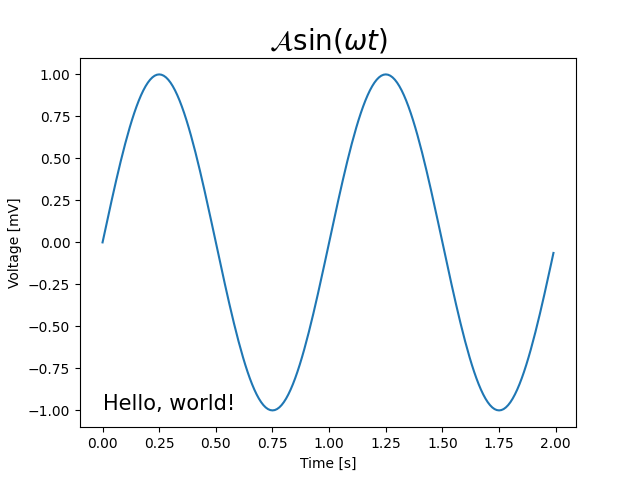# Text and mathtext using pyplot#

Set the special text objects title, xlabel, and ylabel through the dedicated pyplot functions. Additional text objects can be placed in the axes using text.

You can use TeX-like mathematical typesetting in all texts; see also Writing mathematical expressions.

import numpy as np
import matplotlib.pyplot as plt

t = np.arange(0.0, 2.0, 0.01)
s = np.sin(2*np.pi*t)

plt.plot(t, s)
plt.text(0, -1, r'Hello, world!', fontsize=15)
plt.title(r'$\mathcal{A}\sin(\omega t)$', fontsize=20)
plt.xlabel('Time [s]')
plt.ylabel('Voltage [mV]')
plt.show()References

The use of the following functions, methods, classes and modules is shown in this example:

Gallery generated by Sphinx-Gallery